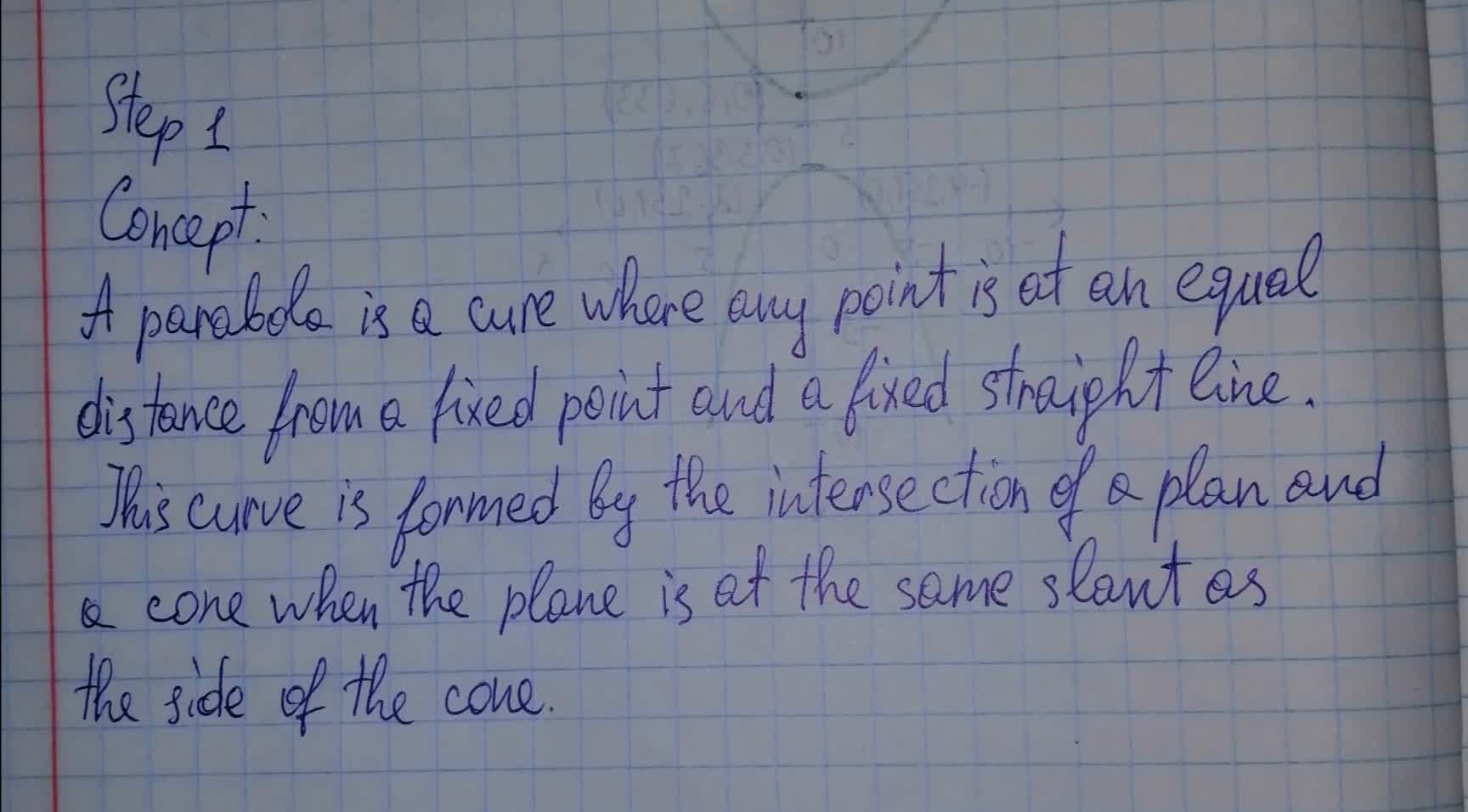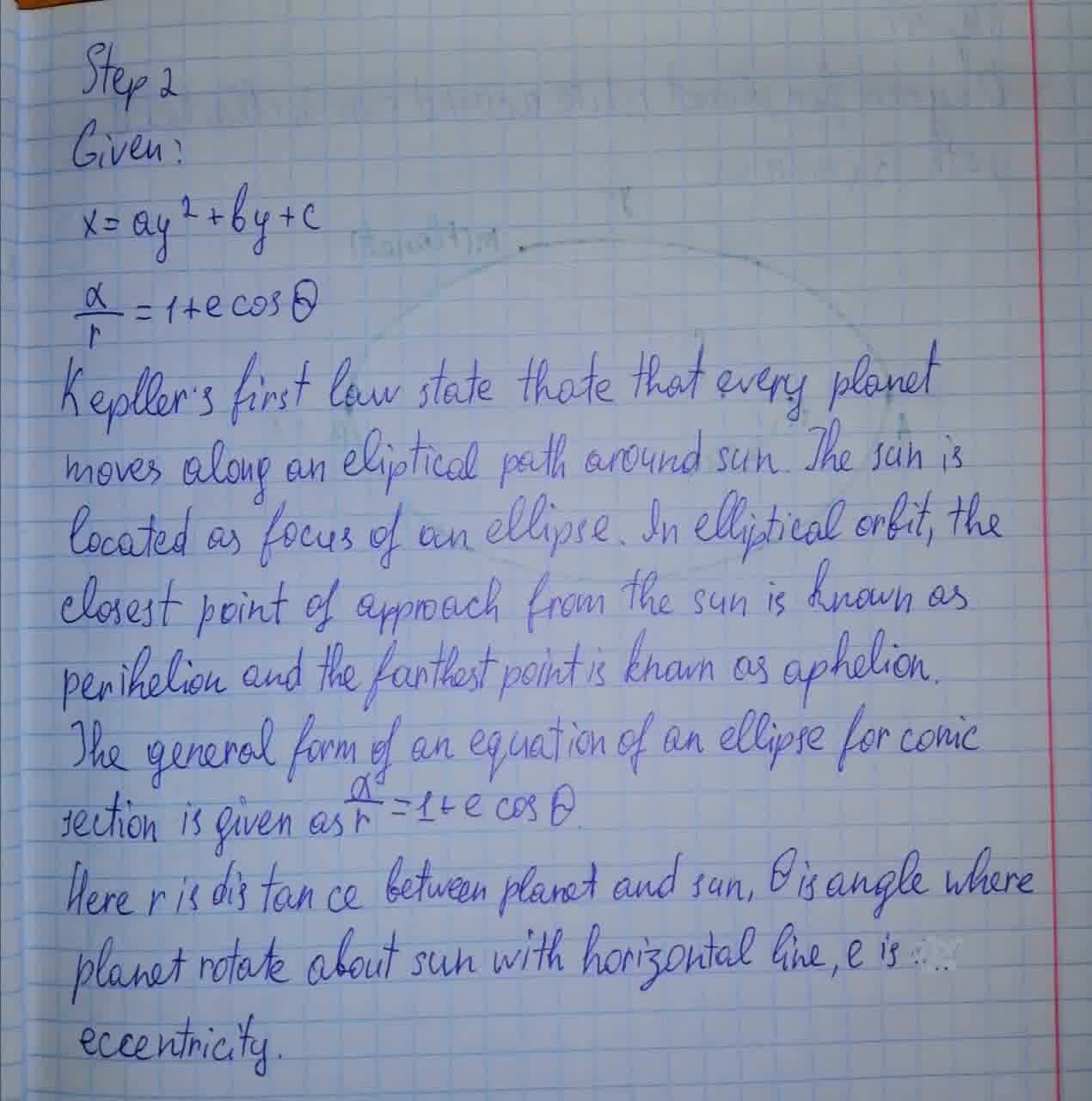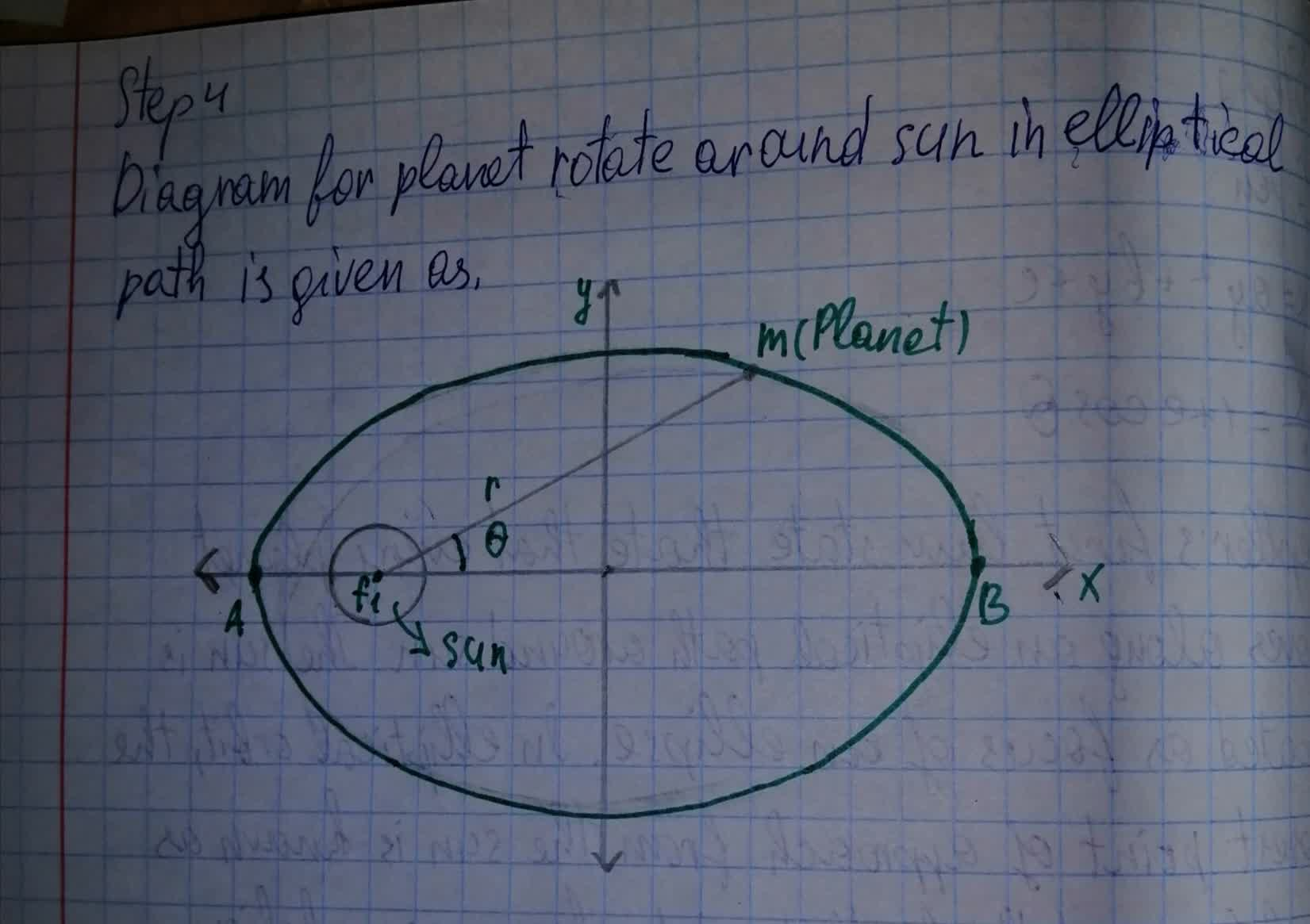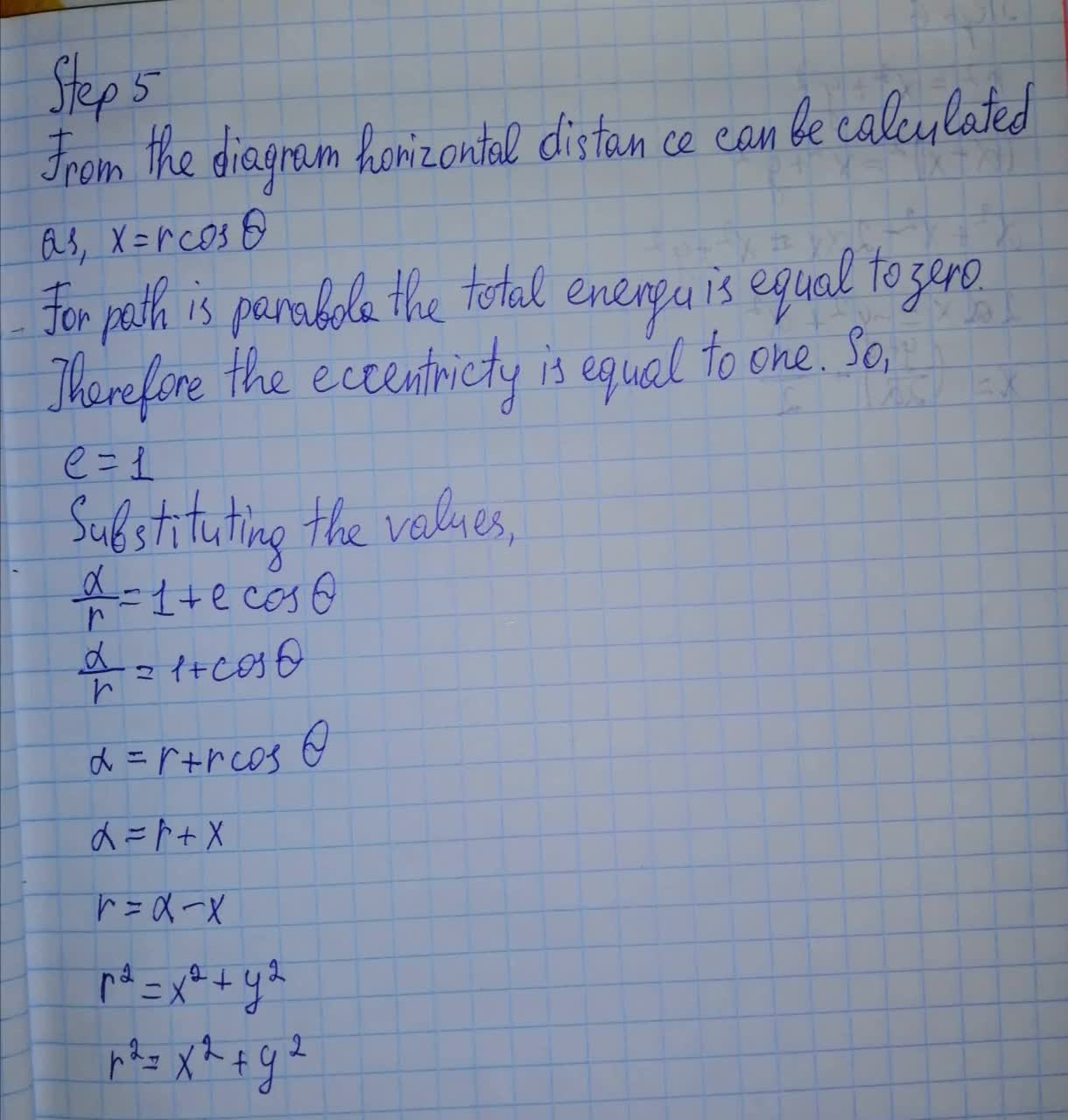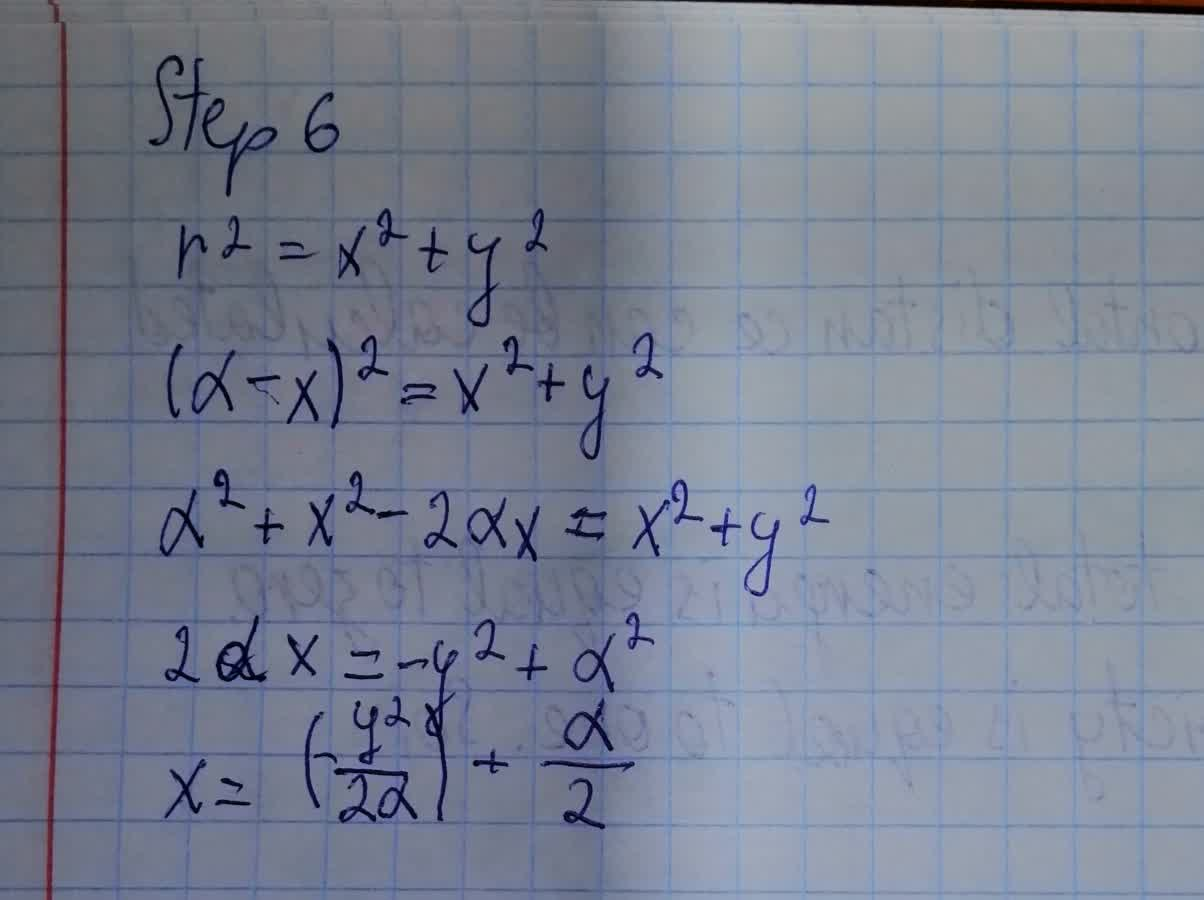Do this by substituting Cartesian coordinates, x and y, for the polar coordinates, r and θ,showing that it has form for a parabola, x = ay^2 + by + cTahmid Knox 2021-08-12 Answered
Show that for eccentricity equal to one in Equation 13.10 for conic sections, the path is a parabola. Do this by substituting Cartesian coordinates, x and y, for the polar coordinates, r and θ , and showing that it has the general form for a parabola, $$\displaystyle{x}={a}{y}^{{2}}+{b}{y}+{c}$$ .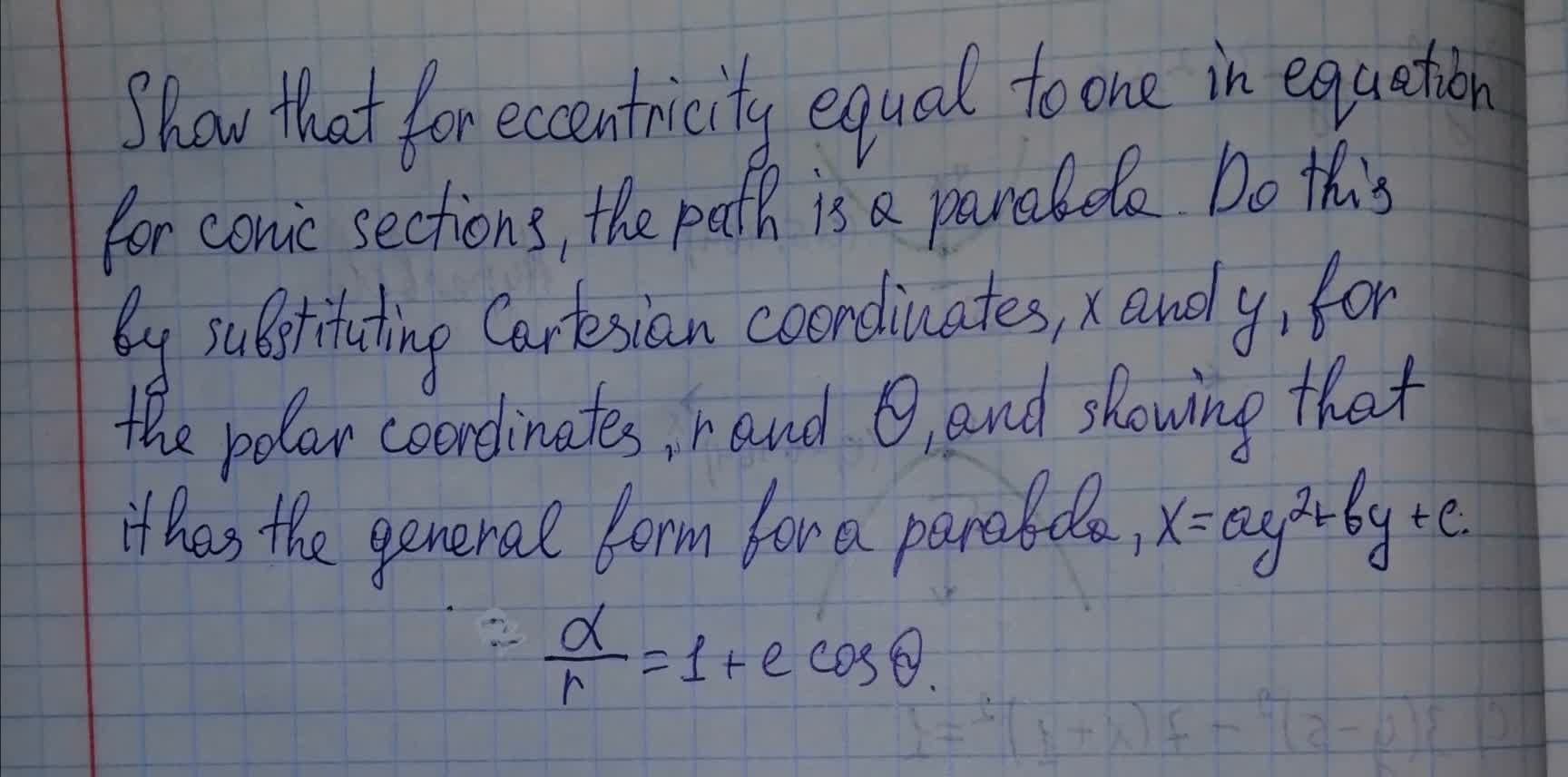• Questions are typically answered in as fast as 30 minutes

Solve your problem for the price of one coffee

• Math expert for every subject
• Pay only if we can solve itUsamah Prosser

Explanation: